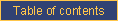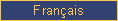homefeaturesDOCUMENTATIONpricingcontact ustechnical documentationCATI documentationcookbookrobotAppendix K

Weights (deprecated)

A WEIGHT question calculates a weighting scheme based on the known marginal distribution of some variables, using the rim weighting algorithm initially proposed by Deming and Stephan (W. Edwards Deming and Frederick F. Stephan, "On a Least Squares Adjustment of a Sampled Frequency Table When the Expected Marginal Totals are Known", The Annals of Mathematical Statistics, volume 11, number 4, December 1940, pp. 427-444). This weighting scheme is stored in the open-end part of the WEIGHT variable; it can be extracted or used in cwfreq to create weighted frequency tables.

Weighting instructions are placed in the question text segment of the question. Each weighting question is listed followed by the weight for each value of the question separated by slashes and ordered according to the numerical list of categories. Questions are separated by commas. Here is an example of the syntax:

QWEIGHT WEIGHT
%
QSEX=0.5/0.5/0
QLANGUAGE = 0.80 / 0.08 / 0.12 / 0 / 0

Weight calculation supports the following (cwnav) options:

• the calculation can be "prudent" which means that all cases with missing data (e.g., "don't-know" category which get a weight of zero) on any variable get a weight of 1; it can also use "maximum information" which means that cases with partial missing data are assigned a weight based on the available data;
• the number of iterations performed can be set by the user (default 10). As a result of the calculation, the root mean square value of the difference between the target numbers and the weighted numbers is displayed at each iteration of weighting. Ideally, this value should approach 0.01 for effective weights.
• the calculation can be limited to a subset of cases using the case selection tools available in all utility programs.

The following rules are enforced:

• WEIGHT variables must have an open-end part;
• all questions referenced in the WEIGHT definition must exist;
• all weights for one question must total 1;
• all weights must be expressed using a decimal point rather than a comma;
• there must be one weight for each defined value of the variable; some weights can be set to zero (e.g., "don't-know" category).

Weights are calculated in cwnav by selecting from the WEIGHT variable list shown after the write-access password is entered.

Weights are used in cwfreq by selecting from the WEIGHT variable list offered.e-mail technical support!